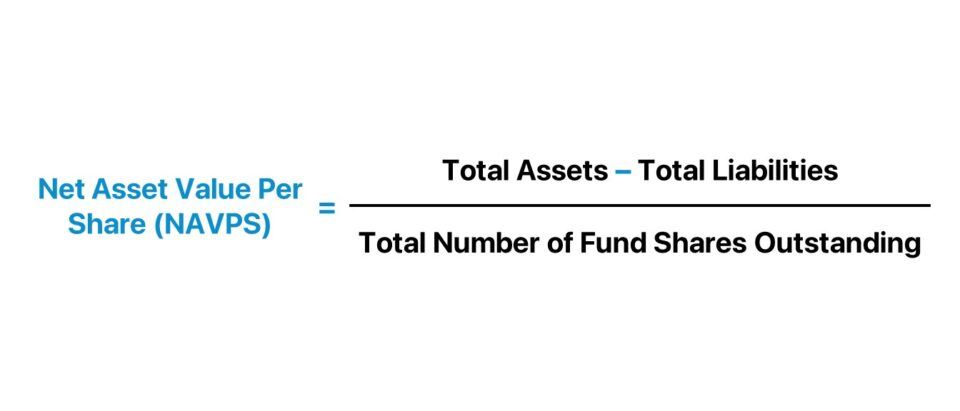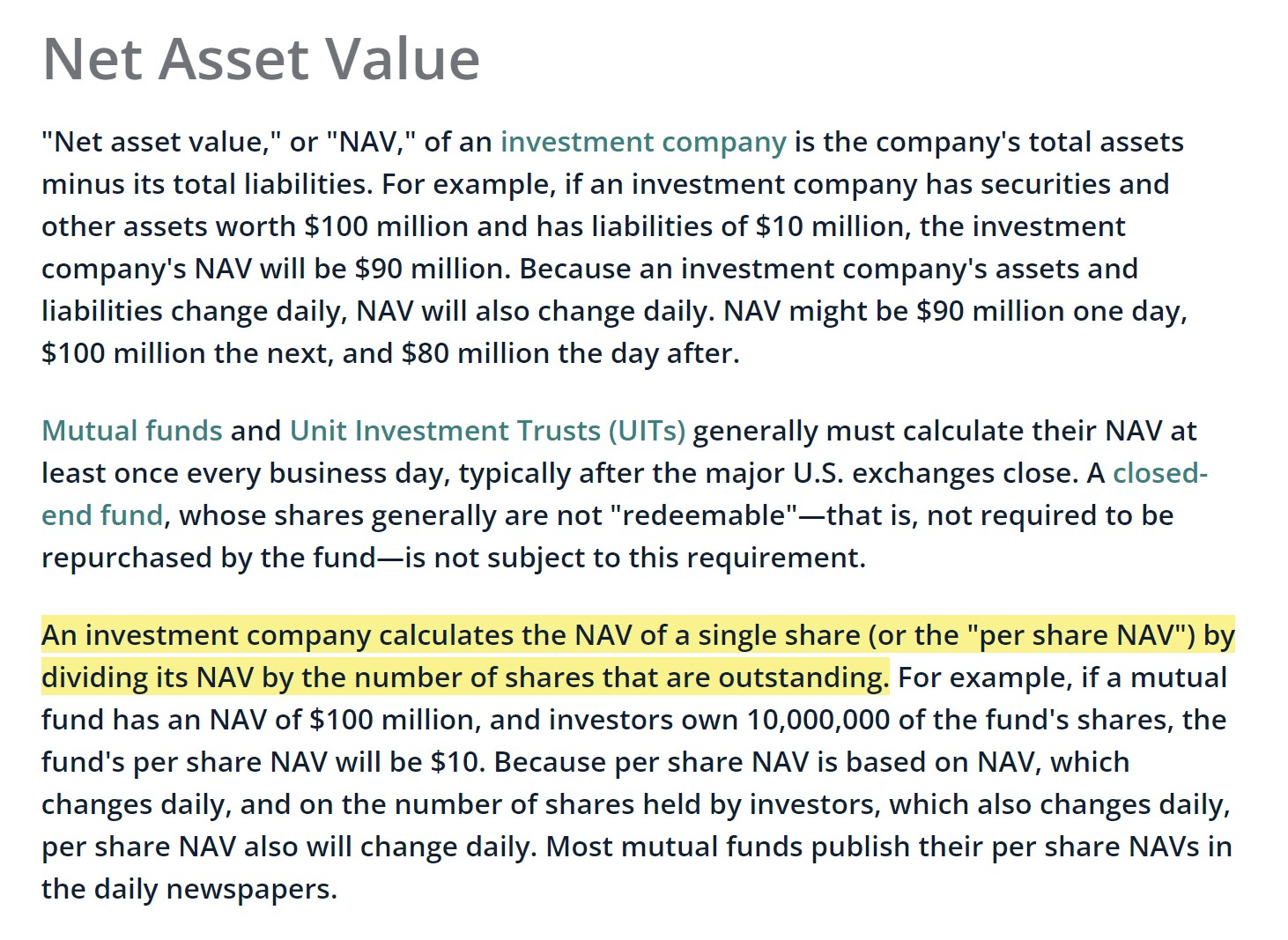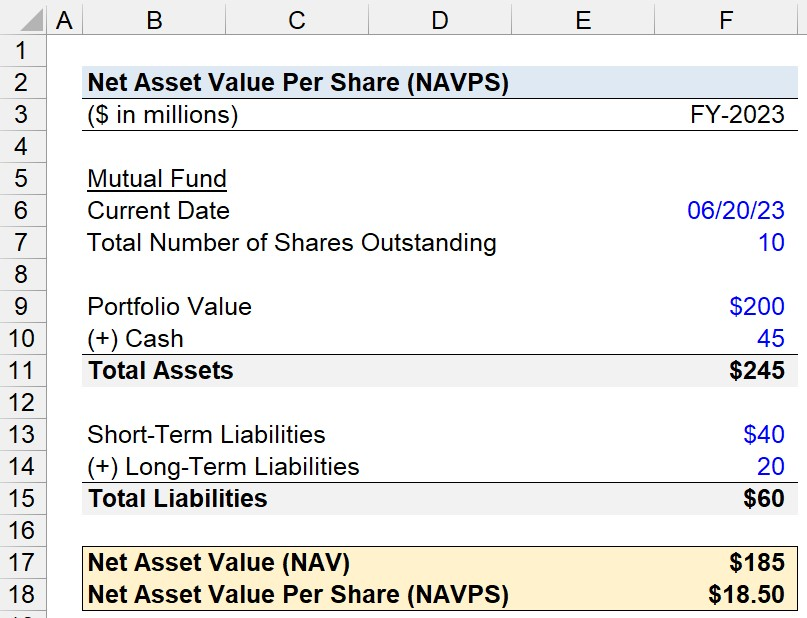Welcome to Wall Street Prep! Use code at checkout for 15% off.# NAV Per Share (NAVPS)

Guide to Understanding NAV Per Share (NAVPS)## How to Calculate NAV Per Share?

In the context of mutual funds, the net asset value (NAV) is the current market value of the fund’s portfolio and others assets, net of any liabilities.

In short, the net asset value (NAV) is the difference between the value of a fund’s assets and liabilities.

Net Asset Value (NAV) = Fund Assets Fund Liabilities

From there, the NAV per share (NAVPS) can be determined by dividing the net asset value of a fund by the total number of shares (or units) outstanding.

For the investment community, such as investors in mutual funds, the NAV per share is a method to monitor the performance of a given fund.

Because of the regulations and oversight by the SEC, mutual funds must provide full transparency with regard to performance and publicly disclose the current value of their portfolio to investors every trading date.NAV Per Share Definition (Source: SEC Glossary)

## NAV Per Share Formula (NAVPS)

The formula to calculate the NAV per share is as follows.

Net Asset Value (NAV) = (Total Assets Total Liabilities) ÷ Total Fund Shares Outstanding

The “Total Assets Total Liabilities” component represents the mutual fund’s net asset value (NAV), while dividing by the total number of shares outstanding of the fund converts the fund’s NAV into a metric expressed on a per-share basis.

## NAV Per Share Calculator

We’ll now move on to a modeling exercise, which you can access by filling out the form below.#### Get the Excel Template!Submitting...

## Mutual Fund NAV Per Share Calculation Example

Suppose you’re tasked with calculating the net asset value per share (NAVPS) of a mutual fund as of its latest closing date (06/20/23).

On the latest reporting date of the mutual fund, the fund’s portfolio has a market value of \$200 million, with \$45 million in cash on the assets side.

• Portfolio Value = \$200 million
• Cash = \$45 million
• Total Assets = \$200 million + 45 million = \$245 million

On the liabilities side, the mutual fund has \$40 million and \$20 million in short-term and long-term liabilities, respectively.

• Short-Term Liabilities = \$40 million
• Long-Term Liabilities = \$20 million
• Total Liabilities = \$40 million + \$20 million = \$60 million

Thus, the mutual fund’s balance sheet carrying values have a total assets balance of \$245 million, while its total liabilities are \$60 million.

By subtracting the mutual fund’s total liabilities from its total assets, the fund’s net asset value (NAV) amounts to \$185 million.

• Net Asset Value (NAV) = \$245 million – \$60 million = \$185 million

Our final assumption is the total number of shares outstanding (i.e. units) of the mutual fund, which we’ll assume to be 10 million.

• Total Number of Shares Outstanding = 10 million

Upon dividing the net asset value (NAV) of the mutual fund by the total number of shares outstanding, we arrive at a NAV per share (NAVPS) of \$18.50.

• NAV Per Share = \$185 million ÷ 10 million = \$18.50Step-by-Step Online Course

### Learn Buy-Side (Hedge Fund) Modeling

Led by a former hedge fund PM (Maverick, Citadel, DE Shaw, Schonfeld), this program begins where financial modeling training ends — with a deep-dive into how buy-side analysts build financial models to make key investment decisions.

Inline Feedbacks